Foxtable(狐表)用户栏目专家坐堂 → 帮我看看哪里错了？

共有391人关注过本帖树形打印复制链接

# 主题：帮我看看哪里错了？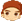hongye
1楼 | 信息 | 搜索 | 邮箱 | 主页 | UC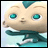加好友发短信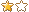Dim sa1 As String = "A01"
Dim fsc As String = "开piao数据"
Dim drU As DataRow
drU = DataTables("表C").Find("[执行编号] = '" & sa1 & "' And [分类] = '" & fsc & "'")
Dim dzml1 As String = drU("主文件夹目录")
Dim dzml2 As String = drU("次文件夹目录")
For Each f As String In FileSys.GetFiles(dzml2)
If f.EndsWith(".log") Then
Dim txt As String = FileSys.ReadAllText(f, Encoding.Default)
Dim xmlDoc As New System.XML.XmlDocument
xmlDoc.Loadxml(txt.Trim)
Dim ndList  = xmlDoc.GetElementsByTagName("comment")
For Each dr As object In ndlist
Dim fpdm As String
Dim fphm As String
Dim ghdwmc As String
Dim ghdwdzdh As String
Dim ghdwsbh As String
Dim ghdwyhzh As String
Dim hjje As String
Dim hjse As String
Dim jshj As String
Dim bz As String
For i As Integer = 0 To ndList.count - 1
If ndList(i).Item("fpdm") IsNot Nothing Then fpdm = ndList(i).Item("fpdm").InnerText
If ndList(i).Item("fphm") IsNot Nothing Then fphm = ndList(i).Item("fphm").InnerText
If ndList(i).Item("ghdwmc") IsNot Nothing Then ghdwmc = ndList(i).Item("ghdwmc").InnerText
If ndList(i).Item("ghdwdzdh") IsNot Nothing Then ghdwdzdh = ndList(i).Item("ghdwdzdh").InnerText
If ndList(i).Item("ghdwsbh") IsNot Nothing Then ghdwsbh = ndList(i).Item("ghdwsbh").InnerText
If ndList(i).Item("ghdwyhzh") IsNot Nothing Then ghdwyhzh = ndList(i).Item("ghdwyhzh").InnerText
If ndList(i).Item("hjje") IsNot Nothing Then hjje = ndList(i).Item("hjje").InnerText
If ndList(i).Item("hjse") IsNot Nothing Then hjse = ndList(i).Item("hjse").InnerText
If ndList(i).Item("jshj") IsNot Nothing Then jshj = ndList(i).Item("jshj").InnerText
If ndList(i).Item("bz") IsNot Nothing Then bz = ndList(i).Item("bz").InnerText
Next
Dim ndList1  = xmlDoc.GetElementsByTagName("fyxm")(0).ChildNodes
For i As Integer = 0 To ndList.count - 1
Dim spmc As String = ndList1(i).Item("spmc").InnerText
Dim ggxh As String = ndList1(i).Item("ggxh").InnerText
Dim sl As String = ndList1(i).Item("sl").InnerText
Dim se As String = ndList1(i).Item("se").InnerText
Dim dw As String = ndList1(i).Item("dw").InnerText
Dim spsl As String = ndList1(i).Item("spsl").InnerText
Dim dj As String = ndList1(i).Item("dj").InnerText
Dim je As String = ndList1(i).Item("je").InnerText
Dim r As Row = Tables("表B").AddNew
r("第一列") = fpdm
r("第二列") = fphm
r("第三列") = ghdwmc
r("第四列") = spmc
r("第五列") = ggxh
r("第六列") = dw
r("第七列") = spsl
r("第八列") = dj
r("第十列") = "str"
Next
Next
End If
Next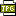此主题相关图片如下：01.jpg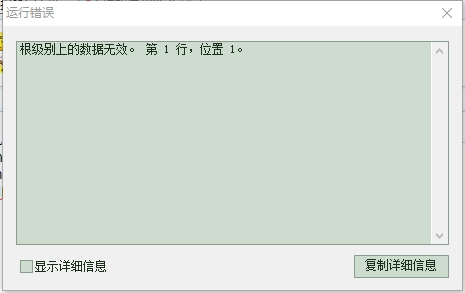下载信息  [文件大小：   下载次数： ]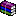点击浏览该文件:_2020-12-19.rar

[此贴子已经被作者于2021/3/22 14:51:03编辑过]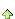2楼 | 信息 | 搜索 | 邮箱 | 主页 | UC加好友发短信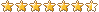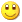Post By：2021/3/22 14:57:00 [只看该作者]

 里面有不属于xml内容的字符，要去掉才能作为xml解析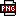此主题相关图片如下：1.png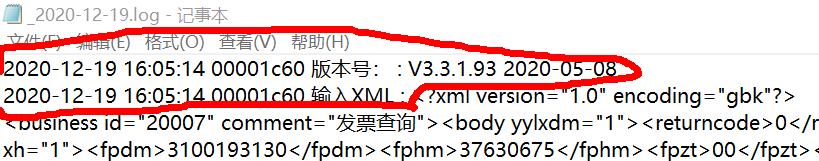hongye
3楼 | 信息 | 搜索 | 邮箱 | 主页 | UC加好友发短信Post By：2021/3/22 14:59:00 [只看该作者]

 那有什么办法读取的时候去掉呢？4楼 | 信息 | 搜索 | 邮箱 | 主页 | UC加好友发短信Post By：2021/3/22 15:01:00 [只看该作者]hongye
5楼 | 信息 | 搜索 | 邮箱 | 主页 | UC加好友发短信Post By：2021/3/22 18:18:00 [只看该作者]此主题相关图片如下：01.jpg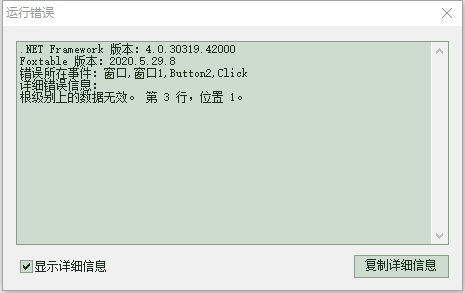现在变这样了6楼 | 信息 | 搜索 | 邮箱 | 主页 | UC加好友发短信Post By：2021/3/22 20:34:00 [只看该作者]hongye
7楼 | 信息 | 搜索 | 邮箱 | 主页 | UC加好友发短信Post By：2021/3/23 7:21:00 [只看该作者]

Dim sa1 As String = "A01"
Dim fsc As String = "开piao数据"
Dim drU As DataRow
drU = DataTables("表C").Find("[执行编号] = '" & sa1 & "' And [分类] = '" & fsc & "'")
Dim dzml1 As String = drU("主文件夹目录")
Dim dzml2 As String = drU("次文件夹目录")
For Each f As String In FileSys.GetFiles(dzml2)
If f.EndsWith(".log") Then
Dim txt As String = FileSys.ReadAllText(f, Encoding.Default)
Dim idx As Integer = txt.IndexOf("<?xml version")
Dim s2 As String = txt.SubString(idx)
Dim sx As String = "s2"
MessageBox.Show(s2)
Dim xmlDoc As New System.XML.XmlDocument
xmlDoc.Loadxml(s2)
MessageBox.Show("0")
Dim ndList  = xmlDoc.GetElementsByTagName("comment")
For Each dr As object In ndlist
Dim fpdm As String
Dim fphm As String
Dim ghdwmc As String
Dim ghdwdzdh As String
Dim ghdwsbh As String
Dim ghdwyhzh As String
Dim hjje As String
Dim hjse As String
Dim jshj As String
Dim bz As String
For i As Integer = 0 To ndList.count - 1
If ndList(i).Item("fpdm") IsNot Nothing Then fpdm = ndList(i).Item("fpdm").InnerText
If ndList(i).Item("fphm") IsNot Nothing Then fphm = ndList(i).Item("fphm").InnerText
If ndList(i).Item("ghdwmc") IsNot Nothing Then ghdwmc = ndList(i).Item("ghdwmc").InnerText
If ndList(i).Item("ghdwdzdh") IsNot Nothing Then ghdwdzdh = ndList(i).Item("ghdwdzdh").InnerText
If ndList(i).Item("ghdwsbh") IsNot Nothing Then ghdwsbh = ndList(i).Item("ghdwsbh").InnerText
If ndList(i).Item("ghdwyhzh") IsNot Nothing Then ghdwyhzh = ndList(i).Item("ghdwyhzh").InnerText
If ndList(i).Item("hjje") IsNot Nothing Then hjje = ndList(i).Item("hjje").InnerText
If ndList(i).Item("hjse") IsNot Nothing Then hjse = ndList(i).Item("hjse").InnerText
If ndList(i).Item("jshj") IsNot Nothing Then jshj = ndList(i).Item("jshj").InnerText
If ndList(i).Item("bz") IsNot Nothing Then bz = ndList(i).Item("bz").InnerText
Next
Dim ndList1  = xmlDoc.GetElementsByTagName("fyxm")(0).ChildNodes
For i As Integer = 0 To ndList.count - 1
Dim spmc As String = ndList1(i).Item("spmc").InnerText
Dim ggxh As String = ndList1(i).Item("ggxh").InnerText
Dim sl As String = ndList1(i).Item("sl").InnerText
Dim se As String = ndList1(i).Item("se").InnerText
Dim dw As String = ndList1(i).Item("dw").InnerText
Dim spsl As String = ndList1(i).Item("spsl").InnerText
Dim dj As String = ndList1(i).Item("dj").InnerText
Dim je As String = ndList1(i).Item("je").InnerText
Dim r As Row = Tables("表B").AddNew
r("第一列") = fpdm
r("第二列") = fphm
r("第三列") = ghdwmc
r("第四列") = spmc
r("第五列") = ggxh
r("第六列") = dw
r("第七列") = spsl
r("第八列") = dj
r("第十列") = "str"
Next
Next
End If
Next
 下载信息  [文件大小：   下载次数： ]点击浏览该文件:_2020-12-19.rar
 下载信息  [文件大小：   下载次数： ]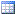点击浏览该文件:xml项目.foxdb

[此贴子已经被作者于2021/3/23 8:31:51编辑过]8楼 | 信息 | 搜索 | 邮箱 | 主页 | UC加好友发短信Post By：2021/3/23 9:19:00 [只看该作者]

 文件里面是N段XML而不是只有一个Dim txt As String = FileSys.ReadAllText("d:\问题\_2020-12-19.log", Encoding.Default)Dim idx As Integer = txt.IndexOf("",idx)Do While idx > -1 And idx2 > -1    Dim s2 As String = txt.SubString(idx,idx2-idx+11)    Output.Show(s2)    Output.Show("-------------------------------")‘对xml的处理    idx  = txt.IndexOf(" -1        idx2  = txt.IndexOf("",idx)    Else        idx2=-1    End IfLoophongye
9楼 | 信息 | 搜索 | 邮箱 | 主页 | UC加好友发短信Post By：2021/3/23 9:57:00 [只看该作者]

 Dim sa1 As String = "A01"Dim fsc As String = "开piao数据"Dim drU As DataRowdrU = DataTables("表C").Find("[执行编号] = '" & sa1 & "' And [分类] = '" & fsc & "'")Dim dzml1 As String = drU("主文件夹目录")Dim dzml2 As String = drU("次文件夹目录")For Each f As String In FileSys.GetFiles(dzml2)    If f.EndsWith(".log") Then        Dim txt As String = FileSys.ReadAllText(f, Encoding.Default)        Dim idx As Integer = txt.IndexOf("",idx)        Do While idx > -1 And idx2 > -1            Dim s2 As String = txt.SubString(idx,idx2-idx+11)            idx  = txt.IndexOf(" -1                idx2  = txt.IndexOf("",idx)            Else                idx2=-1            End If            Dim xmlDoc As New System.XML.XmlDocument            xmlDoc.Loadxml(s2)            Dim ndList  = xmlDoc.GetElementsByTagName("comment")            For Each dr As object In ndlist                Dim fpdm As String                Dim fphm As String                Dim ghdwmc As String                Dim ghdwdzdh As String                Dim ghdwsbh As String                Dim ghdwyhzh As String                Dim hjje As String                Dim hjse As String                Dim jshj As String                Dim bz As String                For i As Integer = 0 To ndList.count - 1                    If ndList(i).Item("fpdm") IsNot Nothing Then fpdm = ndList(i).Item("fpdm").InnerText                    If ndList(i).Item("fphm") IsNot Nothing Then fphm = ndList(i).Item("fphm").InnerText                    If ndList(i).Item("ghdwmc") IsNot Nothing Then ghdwmc = ndList(i).Item("ghdwmc").InnerText                    If ndList(i).Item("ghdwdzdh") IsNot Nothing Then ghdwdzdh = ndList(i).Item("ghdwdzdh").InnerText                    If ndList(i).Item("ghdwsbh") IsNot Nothing Then ghdwsbh = ndList(i).Item("ghdwsbh").InnerText                    If ndList(i).Item("ghdwyhzh") IsNot Nothing Then ghdwyhzh = ndList(i).Item("ghdwyhzh").InnerText                    If ndList(i).Item("hjje") IsNot Nothing Then hjje = ndList(i).Item("hjje").InnerText                    If ndList(i).Item("hjse") IsNot Nothing Then hjse = ndList(i).Item("hjse").InnerText                    If ndList(i).Item("jshj") IsNot Nothing Then jshj = ndList(i).Item("jshj").InnerText                    If ndList(i).Item("bz") IsNot Nothing Then bz = ndList(i).Item("bz").InnerText                Next                Dim ndList1  = xmlDoc.GetElementsByTagName("fyxm")(0).ChildNodes                For i As Integer = 0 To ndList.count - 1                    Dim spmc As String = ndList1(i).Item("spmc").InnerText                    Dim ggxh As String = ndList1(i).Item("ggxh").InnerText                    Dim sl As String = ndList1(i).Item("sl").InnerText                    Dim se As String = ndList1(i).Item("se").InnerText                    Dim dw As String = ndList1(i).Item("dw").InnerText                    Dim spsl As String = ndList1(i).Item("spsl").InnerText                    Dim dj As String = ndList1(i).Item("dj").InnerText                    Dim je As String = ndList1(i).Item("je").InnerText                    Dim r As Row = Tables("表B").AddNew                    r("第一列") = fpdm                    r("第二列") = fphm                    r("第三列") = ghdwmc                    r("第四列") = spmc                    r("第五列") = ggxh                    r("第六列") = dw                    r("第七列") = spsl                    r("第八列") = dj                    r("第十列") = "str"                Next            Next        Loop    End IfNext错误是没有了，但是没有将数据写入表中，这是为什么？10楼 | 信息 | 搜索 | 邮箱 | 主页 | UC加好友发短信Post By：2021/3/23 10:02:00 [只看该作者]

 这个您要自己调试分析xml的节点                Dim ndList1  = xmlDoc.GetElementsByTagName("fyxm")(0).ChildNodesmsgbox(ndList.count )                For i As Integer = 0 To ndList.count - 1                    Dim spmc As String = ndList1(i).Item("spmc").InnerTextmsgbox(spmc  )                    Dim ggxh As String = ndList1(i).Item("ggxh").InnerTextmsgbox(ggxh )……总数 28 1 2 3 下一页